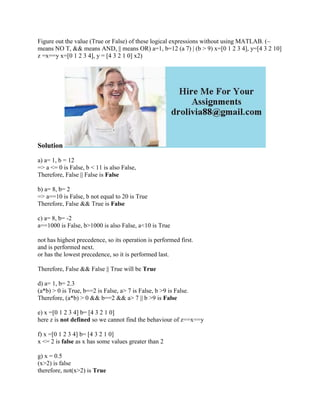Se está descargando tu SlideShare. ×

# Figure out the value (True or False) of these logical expressions with.docx

Anuncio
Anuncio
Anuncio
Anuncio
Anuncio
Anuncio
Anuncio
Anuncio
Anuncio
Anuncio
AnuncioCargando en…3
×

1 de 2 Anuncio

# Figure out the value (True or False) of these logical expressions with.docx

Figure out the value (True or False) of these logical expressions without using MATLAB. (~ means NO T, && means AND, || means OR) a=1, b=12 (a 7) | (b > 9) x=[0 1 2 3 4], y=[4 3 2 10] z =x==y x=[0 1 2 3 4], y = [4 3 2 1 0] x2)
Solution
a) a= 1, b = 12
=> a <= 0 is False, b < 11 is also False,
Therefore, False || False is False
b) a= 8, b= 2
=> a==10 is False, b not equal to 20 is True
Therefore, False && True is False
c) a= 8, b= -2
a==1000 is False, b>1000 is also False, a<10 is True
not has highest precedence, so its operation is performed first.
and is performed next.
or has the lowest precedence, so it is performed last.
Therefore, False && False || True will be True
d) a= 1, b= 2.3
(a*b) > 0 is True, b==2 is False, a> 7 is False, b >9 is False.
Therefore, (a*b) > 0 && b==2 && a> 7 || b >9 is False
e) x =[0 1 2 3 4] b= [4 3 2 1 0]
here z is not defined so we cannot find the behaviour of z==x==y
f) x =[0 1 2 3 4] b= [4 3 2 1 0]
x <= 2 is false as x has some values greater than 2
g) x = 0.5
(x>2) is false
therefore, not(x>2) is True
.

Figure out the value (True or False) of these logical expressions without using MATLAB. (~ means NO T, && means AND, || means OR) a=1, b=12 (a 7) | (b > 9) x=[0 1 2 3 4], y=[4 3 2 10] z =x==y x=[0 1 2 3 4], y = [4 3 2 1 0] x2)
Solution
a) a= 1, b = 12
=> a <= 0 is False, b < 11 is also False,
Therefore, False || False is False
b) a= 8, b= 2
=> a==10 is False, b not equal to 20 is True
Therefore, False && True is False
c) a= 8, b= -2
a==1000 is False, b>1000 is also False, a<10 is True
not has highest precedence, so its operation is performed first.
and is performed next.
or has the lowest precedence, so it is performed last.
Therefore, False && False || True will be True
d) a= 1, b= 2.3
(a*b) > 0 is True, b==2 is False, a> 7 is False, b >9 is False.
Therefore, (a*b) > 0 && b==2 && a> 7 || b >9 is False
e) x =[0 1 2 3 4] b= [4 3 2 1 0]
here z is not defined so we cannot find the behaviour of z==x==y
f) x =[0 1 2 3 4] b= [4 3 2 1 0]
x <= 2 is false as x has some values greater than 2
g) x = 0.5
(x>2) is false
therefore, not(x>2) is True
.

Anuncio
Anuncio

### Figure out the value (True or False) of these logical expressions with.docx

1. 1. Figure out the value (True or False) of these logical expressions without using MATLAB. (~ means NO T, && means AND, || means OR) a=1, b=12 (a 7) | (b > 9) x=[0 1 2 3 4], y=[4 3 2 10] z =x==y x=[0 1 2 3 4], y = [4 3 2 1 0] x2) Solution a) a= 1, b = 12 => a <= 0 is False, b < 11 is also False, Therefore, False || False is False b) a= 8, b= 2 => a==10 is False, b not equal to 20 is True Therefore, False && True is False c) a= 8, b= -2 a==1000 is False, b>1000 is also False, a<10 is True not has highest precedence, so its operation is performed first. and is performed next. or has the lowest precedence, so it is performed last. Therefore, False && False || True will be True d) a= 1, b= 2.3 (a*b) > 0 is True, b==2 is False, a> 7 is False, b >9 is False. Therefore, (a*b) > 0 && b==2 && a> 7 || b >9 is False e) x =[0 1 2 3 4] b= [4 3 2 1 0] here z is not defined so we cannot find the behaviour of z==x==y f) x =[0 1 2 3 4] b= [4 3 2 1 0] x <= 2 is false as x has some values greater than 2 g) x = 0.5 (x>2) is false therefore, not(x>2) is True